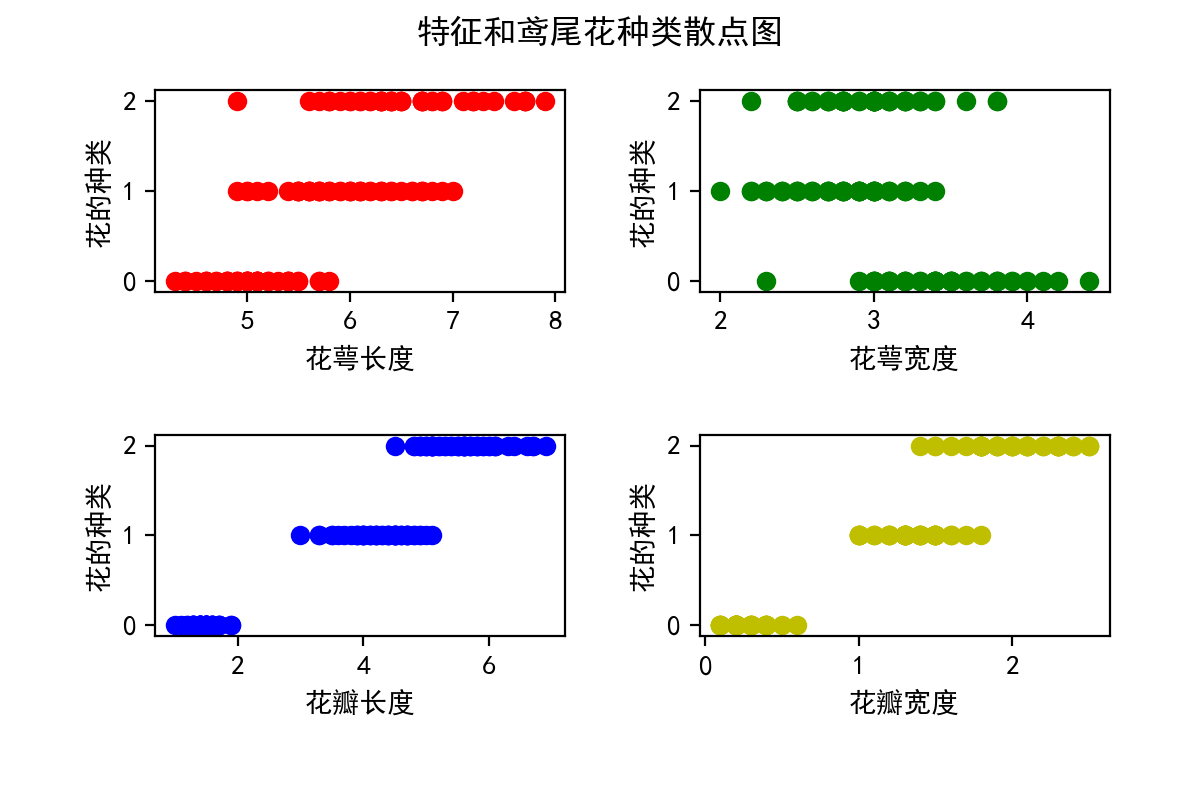03 March 2018

%matplotlib notebook
import numpy as np
import matplotlib.pyplot as plt

# 加载数据集

# 数据特征：150行， 4列
features = iris['data']

# 对应的鸢尾花种类: 150个，三种鸢尾花分别用 0,1,2 表示
target = iris['target']

# 4个特征的名称
feature_names = iris.feature_names
# ['sepal length (cm)', 'sepal width (cm)', 'petal length (cm)', 'petal width (cm)']
#   花萼长度，花萼宽度，花瓣长度，花瓣宽度
feature_names = ['花萼长度', '花萼宽度', '花瓣长度', '花瓣宽度']

# 三种鸢尾花的名称
class_names = iris.target_names
# ['setosa', 'versicolor', 'virginica']
# 山鸢尾花, 变色鸢尾花, 维吉尼亚鸢尾花
class_names = ['山鸢尾花', '变色鸢尾花', '维吉尼亚鸢尾花']


## 信息熵

# 三种鸢尾花数量
iris_count = np.zeros(3)

iris_count = target[target == 0].size
iris_count = target[target == 1].size
iris_count = target[target == 2].size

print("三种鸢尾花的数量分别为：", iris_count)

# 计算每种鸢尾花的概率
iris_probability = np.divide(iris_count, 150)

print("三种莺尾花的概率为：", iris_probability)

# 根据公式算出鸢尾花的熵：
iris_h = -np.sum(iris_probability * np.log2(iris_probability))

print("鸢尾花的熵为：", iris_h)


三种鸢尾花的数量分别为： [50. 50. 50.]



colors='rgby'

# 设置图像大小及dpi
# plt.rcParams["figure.figsize"] = [6, 4]
# plt.rcParams['figure.dpi'] = 100

# 生成散点图
for i in range(4):
plt.subplot(2, 2, i+1)
plt.scatter(features[:,i], target, c=colors[i])
plt.xlabel(feature_names[i])
plt.ylabel('花的种类')

plt.suptitle("特征和鸢尾花种类散点图")
plt.show()## 条件熵

# 定义计算熵公式的函数
def calcEntropy(target):
label = np.unique(target)
n = label.size
count = np.zeros(n)
p_i = np.zeros(n)
for i in range(n):
count[i] = target[target == label[i]].size

# 计算每个类别的概率
p_i = np.divide(count, target.size)

# 计算熵
entropy = 0
for i in range(n):
entropy = entropy - p_i[i] * np.log2(p_i[i])

return entropy

# 定义二分类条件熵公式的函数，把属性的值分为两类
# 对于花瓣宽度来说: 一类是大于 0.8， 另一类就是小于等于 0.8
def calcConditionEntropy(feature, condition, target):
true_condition = condition(feature)
false_condition = true_condition == False
target_true = target[true_condition]
target_false = target[false_condition]
# 每种属性类别的数量除以总数就计算出其概率
p_true = target_true.size / target.size
p_false = 1 - p_true
# 每种属性类别的概率乘以该类别下的信息熵
entropy = p_true * calcEntropy(target_true) + p_false * calcEntropy(target_false)
return entropy

# 重新使用函数计算一次鸢尾花的熵，结果跟上面一样
H = calcEntropy(target)

# 加入鸢尾花花瓣宽度属性后，计算鸢尾花的条件熵
petal_width = features[:,3]
HC = calcConditionEntropy(petal_width, lambda feature: feature < 0.8, target)
print('鸢尾花默认的信息熵 ：', H)
print('带花瓣宽度的条件熵 ：', HC)

鸢尾花默认的信息熵 ： 1.584962500721156



## 信息增益

• 遍历所有特征的信息增益，选择信息增益最大的特征作为根节点的特征
• 由节点特征的不同分类建立子节点，再对各个子节点上述方法选择各子节点的特征
• 重复以上步骤直至所有特征的信息增益都很小或者没有特征可以选择为止

## 特征分类方法

• 对特征的取值进行排序（feature 和 target 需要同步排序）
• 将 target 有变动的地方作为可能的划分点（比例，target取值从0变成1）
• 分界点定义为划分点的对应的两个特征取值的平均值
• 计算所有划分点的信息增益，选取最大的那个信息增益对应的值作为最终选择的分界点

def generate_feature_points(feature, target):
"""
生成特征的所有分界点: 先对特征进行排序，然后将 target 有变动的地方作为分界点
:param feature: 一维数组，一个特征的样本数据
:param target: 一维数组，数字或者字符串的分类标签
:return: 包含所有分界点的一维数组
"""

argsort = feature.argsort()
f1 = feature[argsort]
t1 = target[argsort]

last_value = target
split_value = []

# 找出所有分裂点
for i in range(t1.size):
if last_value != t1[i]:
split_value.append((f1[i] + f1[i - 1]) / 2)
last_value = t1[i]

return np.array(split_value)

def calc_feature_entropy(feature, target):
"""
计算一个特征的所有分界点的条件熵，返回最小的那个条件熵（条件熵越小，信息增益越大）
:param feature: 一维数组，一个特征的样本数据
:param target: 一维数组，数字或者字符串的分类标签
:return: 分界点和条件熵
"""
min_entropy = float('inf')
min_point = 0
points = generate_feature_points(feature, target)
for p in points:
entropy = calcConditionEntropy(feature, lambda f: f < p, target)
if entropy < min_entropy:
min_entropy = entropy
min_point = p

'没有分界点说明只有一类数据标签，熵为0'
if points.size == 0:
min_entropy = 0

return min_point, min_entropy

calc_feature_entropy(features[:,3], target)

(0.8, 0.6666666666666667)


def select_feature(features, target):
"""
从所有特征中选择出条件熵最小的特征（即最大增益）
:param features: 二维数据，包含所有特征的样本数据
:param target: 一维数组，数字或者字符串的分类标签
:return: 特征索引，条件熵，特征分界点
"""
min_entropy = float('inf')
min_point = 0
num = features.shape
index = 0
for i in range(num):
point, entropy = calc_feature_entropy(features[:, i], target)
if entropy <= min_entropy:
index = i
min_point = point
min_entropy = entropy

return index, min_point, min_entropy

class TreeNode:
idn = 0
feature_index = ''
feature_point = 0
feature_entropy = 0
target_label = ''
true_node = None
false_node = None

@staticmethod
def decision(feature, point):
return feature < point

def build_tree(features, target, idn):
"""
递归构建决策树
:param features: 二维数据，包含所有特征的样本数据
:param target: 一维数组，数字或者字符串的分类标签
:param idn: 决策树节点 id，通过 id 观察决策树计算过程
:return: 决策树根节点
"""
node = TreeNode()

'选择条件熵最小的特征'
index, point, entropy = select_feature(features, target)
node.idn = idn
node.feature_index = index
node.feature_point = point
node.feature_entropy = entropy
'取出现次数最多的标签作为该特征节点的输出'
node.target_label = target[np.argmax(np.bincount(target))]

print('build tree node id %d, index %d, point %f, entropy %f, label %s ' %
(idn, index, point, entropy, node.target_label))

'熵小于 0.1 时则结束创建子节点，防止过拟合'
if entropy < 0.1:
print('too low entropy : ', entropy)
return node

f_copy = features.copy()
t_copy = target.copy()
f = f_copy[:, index]
selector = node.decision(f, point)

'创建左右两个子节点'
idn = idn + 1
node.true_node = build_tree(f_copy[selector, :], t_copy[selector], idn)
idn = node.true_node.idn + 1
node.false_node = build_tree(f_copy[selector == False], t_copy[selector == False], idn)
return node

'构建决策树，节点编号从 1 开始，深度优先递归方式创建树'
build_tree(features, target, 1)

build tree node id 1, index 3, point 0.800000, entropy 0.666667, label 0
build tree node id 2, index 3, point 0.000000, entropy 0.000000, label 0
too low entropy :  0
build tree node id 3, index 3, point 1.750000, entropy 0.309840, label 1
build tree node id 4, index 2, point 4.950000, entropy 0.231894, label 1
build tree node id 5, index 3, point 1.650000, entropy 0.000000, label 1
too low entropy :  0.0
build tree node id 6, index 3, point 1.550000, entropy 0.459148, label 2
build tree node id 7, index 3, point 0.000000, entropy 0.000000, label 2
too low entropy :  0
build tree node id 8, index 2, point 5.450000, entropy 0.000000, label 1
too low entropy :  0.0
build tree node id 5, index 1, point 3.150000, entropy 0.112984, label 2
build tree node id 6, index 3, point 0.000000, entropy 0.000000, label 2
too low entropy :  0
build tree node id 7, index 2, point 4.950000, entropy 0.000000, label 2
too low entropy :  0.0

<__main__.TreeNode at 0x10a375b00>


## 增益率（C4.5算法）

ID3 算法时根据信息增益来选择节点，C4.5 算法则时通过增益率来选择节点：

## 基尼指数（CART算法）

CART（Classification And Regression Tree）是一种很重要的机器学习算法，既可以用来创建分类树，也可以创建回归树。

from sklearn.model_selection import train_test_split
from sklearn import tree

# 把样本分成训练集和测试集两部分, 两者比例为： 7:3
X_train, X_test, y_train, y_test = train_test_split(features, target, test_size=0.3, random_state=42)

# 创建决策树分类器
clf = tree.DecisionTreeClassifier()

# 训练决策树
clf.fit(X=X_train, y=y_train)

# 查看特征比重
print("feature weight : ", clf.feature_importances_)

# 查看决策树评分
print("decision tree score : ", clf.score(X=X_test, y=y_test))


feature weight :  [0.         0.01911002 0.89326355 0.08762643]
decision tree score :  1.0

import graphviz

# 使用 graphviz 出决策树的图
dot_data = tree.export_graphviz(
clf,
out_file=None,
feature_names=feature_names,
class_names=class_names,
filled=True,
rounded=True,
special_characters=True)

graph = graphviz.Source(dot_data)
graph